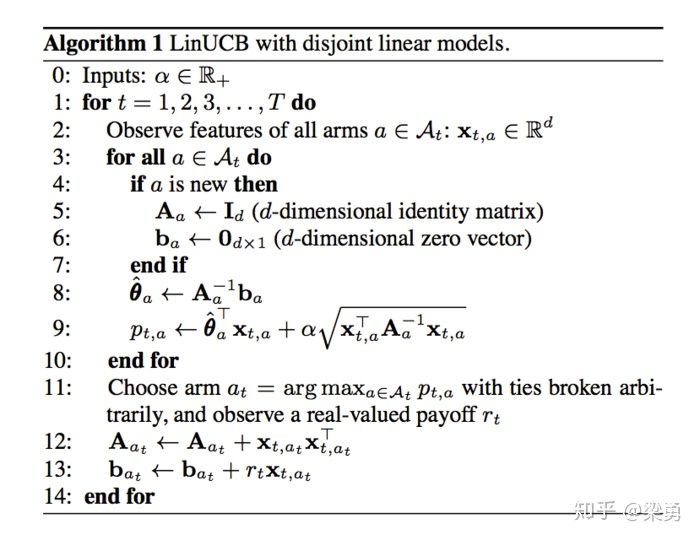# LinUCB算法理解

## 解决的问题

1、UCB的算法context-free：

## 原理

1、每个arm维护一个特征向量：
$\theta_{\alpha}$

2、假设 : 每个arm的期望收益为arm特征向量的线性函数：
$E[r_{t,a}|x_{t,a}] = x_{t,a}^{T}\theta_{a}$

3、这里考虑单个arm上多次实验的总的损失函数：

• m次实验，用户总特征矩阵称为：(D_a), 维度为m * n(用户特征维度)
• m次实验，这个arm前m次的收益为：(c_a)，维度为m*1

$loss = (c_a - D_a\theta_a)^2 + \lambda\theta_a^2$

4、求损失函数的最小值，使损失函数对 (\theta_a) 求导，并且使导数为0，得到：
$\theta_a = (D_a^TD_a + I)^{-1}D_a^Tc_a$

5、为了简洁+选取臂后方便更新数据，将两个乘积分别用A和b表示：
$A = (D_a^TD_a + I)^{-1} \\ b = D_a^Tc_a$

6、上面得到了单个arm使loss最小的参数更新规则，对于多个
arm而言，需要计算每个arm的最大上界，选择最大的arm（老实说，这块公式推导比较麻烦，后期再深入研究）：
$x_{t,a}^T\theta_a + \alpha\sqrt{x_{t,a}^TA_a^{-1}x_{t,a}} \\ 其中\alpha控制选择倾向于Explore还是Exploit\\ \alpha越小，arm的期望收益所占权重越大，选择越倾向于Exploit$

## 实现流程## 优点

• 解决了UCB等MAB算法context-free的问题，考虑到用户的特征和物品的特征
• 计算复杂度同arm的数量成线性关系
• 支持动态变化的候选arm集合，有新的arm出来时，实时支持对新的arm进行初始化，并添加到分数选取中
• 在线学习：在计算arm的参数和recommend的时候，用到了上下文的特征+用户反馈，每次信息
都进行了保存更新到A和b中。A和b可以异步增量更新

## 引用

http://xudongyang.coding.me/linucb/

https://zhuanlan.zhihu.com/p/38875273

©️2019 CSDN 皮肤主题: 大白 设计师: CSDN官方博客